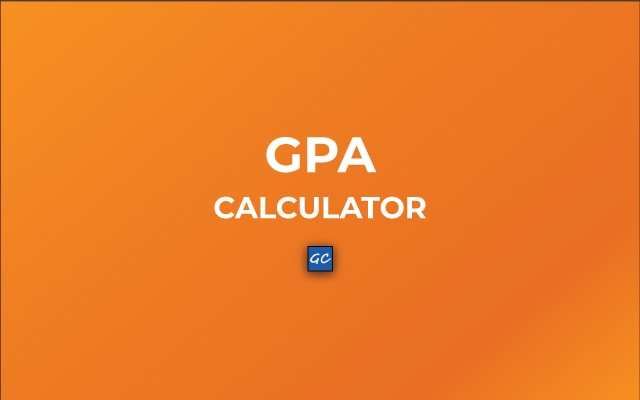# GPA Calculator – Final Grade Point Average Calculator

Use this GPA Calculator to calculate your grade point average online. Just enter the class, grades, and credits/hours. Also, you can find the Final GPA using this.

## Final GPA Calculator

Related### GPA Calculation

GPA is equal to the sum of credit/hours into the grade. You can see the formula below.

GPA = (W1 X G1) + (W2 X G2) + (W3 X G3) + (W4 X G4) + … + (Wn X Gn)

Here,
W = Credit/Hours weight

In addition, we can calculate credit hour weight(Wj) by dividing the credit hours of class(Cj) with the sum of all credit hours classes.

Wj = Cj / (C1 + C2 + C3 + C4 + … + Cn)